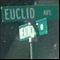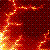Discussion about math, puzzles, games and fun.   Useful symbols: ÷ × ½ √ ∞ ≠ ≤ ≥ ≈ ⇒ ± ∈ Δ θ ∴ ∑ ∫  π  -¹ ² ³ °

You are not logged in.

## #1 2007-11-06 13:01:35

Ricky
ModeratorRegistered: 2005-12-04
Posts: 3,791

### Things they never teach you in algebra

Prove that the following polynomial has no integer roots:

"In the real world, this would be a problem.  But in mathematics, we can just define a place where this problem doesn't exist.  So we'll go ahead and do that now..."

Offline

## #2 2007-11-06 16:54:56

ganeshRegistered: 2005-06-28
Posts: 27,013

### Re: Things they never teach you in algebra

Ricky,
A polynomial of degree 10 is unsolvable by any yardstick!
That's what I think!
To prove that it can be solved, you have to show howI also believe, like Abel did, an equation in one variable of degree more than five, that is a quintic equation, cannot be solved manually! Maybe a supercomputer can do it,and even a supercomputer may take a long time!!!!

It is no good to try to stop knowledge from going forward. Ignorance is never better than knowledge - Enrico Fermi.

Nothing is better than reading and gaining more and more knowledge - Stephen William Hawking.

Offline

## #3 2007-11-07 02:36:14

TheDude
Member
Registered: 2007-10-23
Posts: 361

### Re: Things they never teach you in algebra

We can immediately rule out all integers from 0 to positive infinity.  0 can be ruled out by trial, and it can't be any positive integer since every coefficient is positive.

Looking at the negative integers we can rewrite the equation.  All even powers will remain positive while all negative powers will become negative, so we can rewrite the equation as follows:

Using this new equation we will consider only positive integers for x, which is exactly equivalent to considering only negative integers of x for the original equation.  Now, since the highest power is even we know that the equation will tend to positive infinity as x tends to positive infinity.  What we do now is try, by trial and error, all integers from 1 up to the point that the equation is strictly increasing, at which point we'll be done.

1 - 3 + 15 - 6 + 33 - 45 + 42 - 24 + 3 - 9 + 6 = 13

x = 2:
1024 - 1536 + 3840 - 768 + 2112 - 1440 + 672 - 192 + 12 - 18 + 6 = 3712

For x = 3, we'll look at prime factorizations.  We'll further rewrite the equation as follows:

When x = 3 we can see that the first two terms will cancel out.  The next two terms give us

, which is greater than 0.  Combining the next two terms gives us
, again greater than 0.  The next two terms clearly give us a term greater than 0, since the positive term has both a higher power of 3 and a larger coefficient.  The final 3 terms give us 27 - 27 + 6 = 6.  Since we were able to combine all of the terms to give us either 0 or a positive number we know that the equation is greater than 0 for x = 3.

We will use this same trick to show that all integer values of x greater than 3 result in a positive answer and thus are not roots.

Combine the first two terms to give us

.  Note that this is equivalent to
.  For all x > 3 this is clearly greater than 0, since both x^9 and x - 3 are greater than 0.

Combine the next two terms to give us

.  This term is clearly greater than 0 since the positive term has both a larger coefficient and a larger power than the negative term.

Combine the next 2 terms for

.  Again, this is positive for all x > 3 since 3x^5 and 11x - 15 will both be positive.

Combining the next 2 terms gives us

, which again has a positive term with both a larger power and a larger coefficient, so this is positive.

Finally, combine the final 3 terms for

.  The 6 is obviously positive, and for all x > 3 so will 3x and x - 3.  Therefore, this final term is positive.

Add it all up and we know that the equation is positive for all x > 0, which is equivalent to saying that the original equation is greater than 0 for all x < 0.  Since we also know that 0 and x < 0 are not roots where x is an integer, we know that the equation has no integer roots.

Edit: Holy cow, your answer is way easier than mine.  I don't quite understand how it works, though.  Do you have a proof, or is this a well known theorem?

Last edited by TheDude (2007-11-07 02:45:57)

Wrap it in bacon

Offline

## #4 2007-11-07 05:33:03

JaneFairfax
MemberRegistered: 2007-02-23
Posts: 6,868

### Re: Things they never teach you in algebra

The result assumes that the polynomial is of degree at least 2. (Linear polynomial equations always have solutions.) It also assumes that none of the coefficients (except possibly the leading one) is ±1.

Last edited by JaneFairfax (2007-11-07 05:43:43)

Offline

## #5 2007-11-07 05:37:46

Ricky
ModeratorRegistered: 2005-12-04
Posts: 3,791

### Re: Things they never teach you in algebra

Ganesh, be very careful.  A polynomial of degree greater than 5 can not be solved in genera.  However, many of them can be factored.  For example:

(x - 1)(x-2)(x-3)(x-4)(x-5)(x-6)(x-7)(x-8)(x-9)(x-10)

Multiplying this out gives you a polynomial of degree 10 with roots 1 through 10.

Edit: Holy cow, your answer is way easier than mine.  I don't quite understand how it works, though.  Do you have a proof, or is this a well known theorem?

This is known as Eisenstein's criterion.  It is proved in general for polynomial rings, and can even be applied to a ring's field of fractions.  In this case, we may not only say that my given polynomial has no integer roots and is not reducible in the integers, but also that it is not reducible in the rationals as well (and hence, no rational roots either).   A simple proof can be found on wikipedia.

It also assumes that none of the coefficients (except possibly the leading one) is ±1.

1 is not prime, so the preconditions can't be met if a coefficient is +/- 1

"In the real world, this would be a problem.  But in mathematics, we can just define a place where this problem doesn't exist.  So we'll go ahead and do that now..."

Offline

## #6 2007-11-07 05:57:47

TheDude
Member
Registered: 2007-10-23
Posts: 361

### Re: Things they never teach you in algebra

Very cool, thanks Jane and Ricky

Wrap it in bacon

Offline

## #7 2007-11-07 15:44:15

bossk171
MemberRegistered: 2007-07-16
Posts: 305

### Re: Things they never teach you in algebra

This is great! Ricky (or anyone else) do you have any more "things they never teach you in algebra." I'm looking forward to learning new things like number theory and what not, but I love to expand on my pre-existing knowledge (like Algebra).

Everything TheDude said in his post could be followed by a good algebra II student, and that's what makes it genius, this stuff makes me all warm and fuzzy inside.

Also, TheDude, you have a really sweet sig.

There are 10 types of people in the world, those who understand binary, those who don't, and those who can use induction.

Offline